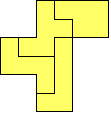Geometric Puzzles in the Classroom# Pentomino Labs

by Henri Picciotto

Slides for a pentomino presentation (grades K-8): PDF | Keynote

Two whole-class challenges, leading to nice bulletin board displays. Both are developed more fully in my book Working with Pentominoes (Where to get it.) However, to carry them out, you need only plastic pentominoes and one-inch grid paper.

## Pentomino Rectangles

A large-scale project for a class is to search for all possible pentomino rectangles (all sizes), and then all possible pentomino rectangle pairs. See Simultaneous Pentomino Rectangles for an account of the number of possible solutions (which you don't have to reveal to your students.)

12-pentomino rectangles are thoroughly discussed in Solomon Golomb's book Polyominoes (Princeton University Press, NJ, 1994.)

## Pentomino Blowups

Another project is to try to cover scaled pentominoes with pentominoes. Print and duplicate the worksheet. Between them, the students should find solutions to as many of the 22 suggested puzzles as possible.

Be sure to discuss questions 2 and 5! This activity alone is not sufficient to establish the relationship between the scaling factor and the ratio of areas, but it helps. For a good introduction to that concept, see Lab 10.3: Polyomino Blowups in my book Geometry Labs.

## Extensions:

• Double duplication: Make a figure, using two pentominoes. Make a congruent copy of that figure, using two other pentominoes. Use the remaining eight pentominoes to make a figure that is similar to the first two. (There are many solutions to this puzzle -- looking for solutions could be a whole-class project.)
• See also the fourth of the superTangram labs on this site.Geometric Puzzles in the Classroom